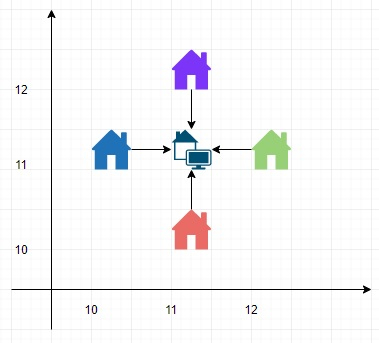# Program to find best position for a service center in Python

PythonServer Side ProgrammingProgramming

#### Beyond Basic Programming - Intermediate Python

Most Popular

36 Lectures 3 hours

#### Practical Machine Learning using Python

Best Seller

91 Lectures 23.5 hours

#### Practical Data Science using Python

22 Lectures 6 hours

Suppose we have a list of positions, which is containing a list of coordinate points where some houses are located. If you want to make a service center at (xc, yc) such that the sum of Euclidean distance from any given point to (xc, yc) is minimum. So we have to find the sum of minimum distance.

So, if the input is like positions = [(10,11),(11,10),(11,12),(12,11)], then the output will be 4.0To solve this, we will follow these steps −

• numIter := 50

• Define a function total() . This will take cx, cy, positions

• total := 0.0

• for each p in positions, do

• x, y := p

• total := total + Euclidean distance between (cx, cy) and (x, y)

• Define a function fy() . This will take x, positions

• l := 0, r := 101

• res := 0

• for i in range 0 to numIter, do

• y1 := l + (r - l) / 3

• y2 := r - (r - l) / 3

• t1 := total(x, y1, positions)

• t2 := total(x, y2, positions)

• res := minimum of t1 and t2

• if t1 < t2, then

• r := y2

• otherwise,

• l := y1

• return res

• Define a function fx() . This will take positions

• l := 0, r := 101

• res := 0

• for i in range 0 to numIter, do

• x1 := l + (r - l) / 3

• x2 := r - (r - l) / 3

• t1 := fy(x1, positions)

• t2 := fy(x2, positions)

• res := minimum of t1 and t2

• if t1 < t2, then

• r := x2

• otherwise,

• l := x1

• return res

From the main method, return fx(positions)

## Example

Let us see the following implementation to get better understanding

from math import sqrt
def solve(points):
numIter = 50

def total(cx, cy, positions):
total = 0.0
for p in positions:
x, y = p
total += sqrt((cx - x) * (cx - x) + (cy - y) * (cy - y))

def fy(x, positions):
l, r = 0, 101
res = 0
for i in range(numIter):
y1 = l + (r - l) / 3
y2 = r - (r - l) / 3
t1 = total(x, y1, positions)
t2 = total(x, y2, positions)
res = min(t1, t2)
if t1 < t2:
r = y2
else:
l = y1
return res

def fx(positions):
l, r = 0, 101
res = 0
for i in range(numIter):
x1 = l + (r - l) / 3
x2 = r - (r - l) / 3
t1 = fy(x1, positions)
t2 = fy(x2, positions)
res = min(t1, t2)
if t1 < t2:
r = x2
else:
l = x1
return res

return fx(positions)

positions = [(10,11),(11,10),(11,12),(12,11)]
print(solve(positions))

## Input

[(10,11),(11,10),(11,12),(12,11)]


## Output

4.0